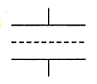# A sheet of aluminium foil of negligible thickness is placed between the plates of a parallel plate capacitor. How will its capacitance be affected if:a)the foil is electrically insulated?b)the coil is connected to the upper plate with the help of connecting wires?7 years ago
1. the capacitor now bcomes like -----|  |  |------ or two caps. in series. with the dist. between them now = d/2 where d= original distance between the plates w/o foil.hence each capacitors now become of cap.=2Coriginal addin up in series they give back a cap.=original cap.hence no effect. bt if the foil wud have been even a bit thick the cap wud have inc. due to intro. of a dielectric with dielectric const.= infinity
2. if the coil is connected to upper plate the cap. becomes 2 times since d=doriginal/2.(hint: the al foil begins to act as a plate nullyfying the effect of upper plate)
6 years ago
The system will be equivalent to two identical capacitors connected in series in which two plates have separation half the original separation. So new capacitance--- c`=2cAs c is inversely proportional to d.So c and c` are in series ==> applying the formula of series combination b/w capacitors we get c which is original capacitor.When foil is connected to upper plate,it reduces to capacitor whose separation reduces to half original one .C`=2xc
2 years ago
) This can be taken as two capacitors in series. The plate separation is d/2 and hence Capacitance is 2C. Total Capacitance is Cs = (2C×2C)/(2C+2C) = C

Hence the Capacitance remain unaffected.

2) d becomes d/2, hence the Capacitance becomes twice the original.